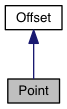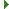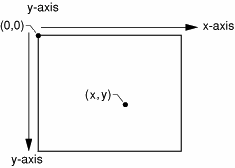PDG engine v0.9.5
Point Class Reference

point for 2D coordinate system More...

Inheritance diagram for Point:[legend]
Collaboration diagram for Point:[legend]

## Public Member Functions

Point ()
create with x = 0, y = 0

Point (number x, number y)
create and set x & y values

Point (number[] xy)
create and set x & y values

Point (object xy)
create and set x & y values

number distance (Point point)
get distance from another point

Point projection (Point point)
do a projection of a second point onto this pointPublic Member Functions inherited from Offset
Offset ()

Offset (number x, number y)

Offset (number[] xy)

Offset (object xy)

Offset add (Offset offset)

Offset assign (Offset offset)

Offset div (Offset offset)

Offset dividedby (Offset offset)

boolean equals (Offset offset)

Offset minus (Offset offset)

Offset mul (Offset offset)

boolean notEquals (Offset offset)

Offset plus (Offset offset)

Offset sub (Offset offset)

Offset times (Offset offset)

## Public Attributes

number x

number yPublic Attributes inherited from Offset
number x

number y

## Detailed Description

point for 2D coordinate system

Point provides support for dealing with points as x, y floating point values in 2 dimensional space. The PDG coordinate space starts with the top left corner of the drawing port at Point(0,0) and the bottom left corner at Point( width, height );## Constructor & Destructor Documentation

 Point ( )

create with x = 0, y = 0

 Point ( number x, number y )

create and set x & y values

Parameters
 x the horizontal coordinate y the vertical coordinate
 Point ( number[] xy )

create and set x & y values

Parameters
 xy an array of numbers with x as element 0 and y as element 1
 Point ( object xy )

create and set x & y values

Parameters
 xy an object with x and y members, for example: {x: 1, y: 4}

## Member Function Documentation

 distance ( Point point )

get distance from another point

Parameters
 point the other Point
 projection ( Point point )

do a projection of a second point onto this point

## Member Data Documentation

 number x
 number y